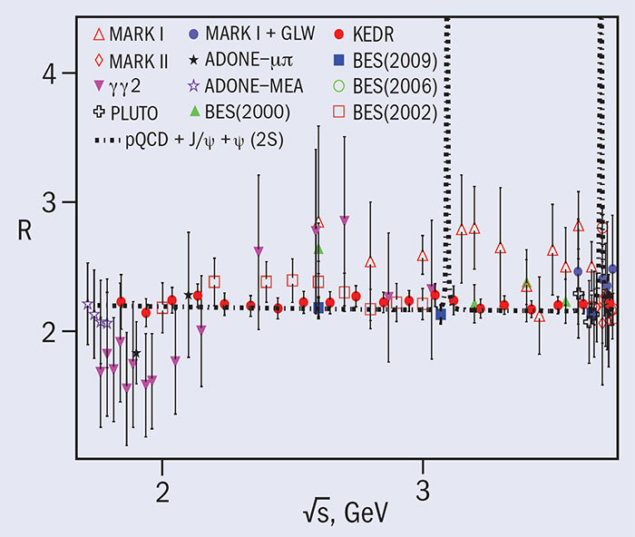# KEDR pins down R at low energies

11 August 2017Measured R value versus the centre-of-mass energy and the sum of the prediction of perturbative QCD and a contribution from narrow resonances.

The KEDR collaboration has used the VEPP-4M electron–positron collider at the Budker Institute in Russia to make the most precise measurement of the quantity “R” in the low-energy range. R is defined as the ratio of the radiatively corrected total hadronic cross-section in electron–positron annihilation to the Born cross-section of muon pair production. The dependence of R on the centre-of-mass energy is critical for determining the running strong coupling constant and heavy-quark masses, the anomalous magnetic moment of the muon and the value of the electromagnetic fine structure constant at the Z peak. A substantial contribution to the uncertainties on these quantities comes from the energy region below charm threshold, where KEDR measurements were made.

The KEDR team performed a precise measurement of R at 20 points: in the energy ranges 1.84–3.05 and 3.12–3.72 GeV the weighted averages of R are 2.225±0.051 and 2.189±0.047, respectively, in good agreement with perturbative QCD. At present, it is the most accurate measurement of R in this energy range, to which more than 10 experiments have contributed. It involved a challenging analysis in which the hadronisation of light quarks at low energies was modelled by tuning distributions of parameters essential for the event selection in the various generator codes.

The collaboration now plans to measure R in the range 5–7 GeV, where the last similar experiment was carried out more than a quarter of a century ago.